Next: 6.1.2 Operation Principle Up: 6.1 Fundamentals Previous: 6.1 Fundamentals

## 6.1.1 Problem Formulation

The state of the EM field is uniquely determined by the Maxwell equations, which hold at every point in whose neighborhood the physical properties of the medium are continuous [11, pp. 1-7]: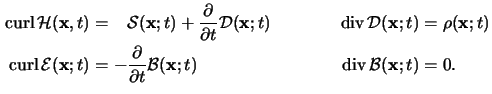These four equations have to be supplemented by material equations also called constitutive relations which describe the behavior of the medium under the influence of the EM field. In our case the materials, i.e., the resist and the nonplanar wafer portions, are isotropic, inhomogeneous, linear and time-invariant.a The constitutive relations thus take the relatively simple form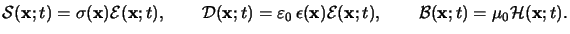(6.1)

Insertion of these relations into the first and second Maxwell equations gives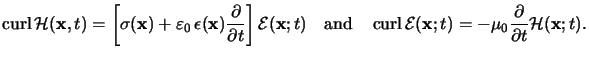(6.2)

As the incident EM disturbance is a time-harmonic one we employ the complex phasor notation already used in previous chapters (cf. (4.1) or (5.22)), i.e.,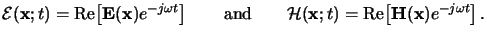(6.3)

Hence the first two Maxwell equations (6.3) write in phasor notation as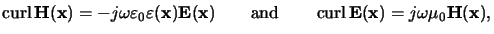(6.4)

whereby the complex-valued relative permittivity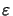(x) is defined by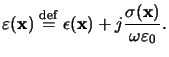(6.5)

The permittivity(x) is simply related to the refractive index n(x) by Maxwell's formula given in (5.23).

The electric and magnetic phasor, E(x) and H(x), respectively, are related by the two coupled, first-order PDEs of (6.5). These PDEs are the time-harmonic versions of the first and second Maxwell equations. The third and fourth Maxwell equations are automatically satisfied by any solution of (6.5) or, respectively, of (6.3). However, an interesting phenomenon follows from the third Maxwell equation. Due to the conductivity of the materials considered a charge density is generated by any EM disturbance incident from outside. In case of a homogeneous conducting material the induced charge density can be neglected as it falls off exponentially with time, whereby the relaxation time is very much shorter than the periodic time of the field vibration [11, pp. 612-615]. In an inhomogeneous conductive material a time-harmonic fluctuation is superposed to this almost instantaneously vanishing transient term. This situation can be accounted for by replacing the real-valued permittivity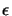(x) in the third Maxwell equation by its complex-valued pendant(x) (cf. (6.6)). Then the third and fourth Maxwell equations take the form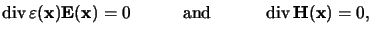(6.6)

and are thus automatically satisfied by any solution of (6.5). They do not carry any additional physical information or importance and thus do not have to be considered separately.

#### Footnotes

... time-invariant.a
The resist is essentially an optically nonlinear material since it changes its properties during exposure. However, the assumptions made throughout the quasi-static approach transform the nonlinear problem to a number of inhomogeneous but linear ones (cf. Section 5.1.4).Next: 6.1.2 Operation Principle Up: 6.1 Fundamentals Previous: 6.1 Fundamentals
Heinrich Kirchauer, Institute for Microelectronics, TU Vienna
1998-04-17